## The Discovery of the Parts of the Atom

Modern scientific usage denotes the atom as composed of constituent particles: the electron, the proton and the neutron.

### Learning Objectives

Discuss experiments that led to discovery of the electron and the nucleus

### Key Takeaways

#### Key Points

• The British physicist J. J. Thomson performed experiments studying cathode rays and discovered that they were unique particles, later named electrons.
• Rutherford proved that the hydrogen nucleus is present in other nuclei.
• In 1932, James Chadwick showed that there were uncharged particles in the radiation he was using. These particles, later called neutrons, had a similar mass of the protons but did not have the same characteristics as protons.

#### Key Terms

• scintillation: A flash of light produced in a transparent material by the passage of a particle.
• alpha particle: A positively charged nucleus of a helium-4 atom (consisting of two protons and two neutrons), emitted as a consequence of radioactivity.
• cathode: An electrode through which electric current flows out of a polarized electrical device.

Though originally viewed as a particle that cannot be cut into smaller particles, modern scientific usage denotes the atom as composed of various subatomic particles. The constituent particles of an atom (each discovered independently) are: the electron, the proton and the neutron. (The hydrogen-1 atom, however, has no neutrons, and a positive hydrogen ion has no electrons. )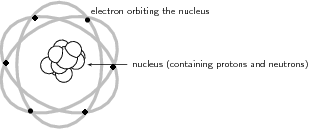Classical Atomic Model: Atomic model before the advent of Quantum Mechanics.

### Electron

The German physicist Johann Wilhelm Hittorf undertook the study of electrical conductivity in rarefied gases. In 1869, he discovered a glow emitted from the cathode that increased in size with decrease in gas pressure. In 1896, the British physicist J. J. Thomson performed experiments demonstrating that cathode rays were unique particles, rather than waves, atoms or molecules, as was believed earlier. Thomson made good estimates of both the charge $\text{e}$ and the mass $\text{m}$, finding that cathode ray particles (which he called “corpuscles”) had perhaps one thousandth the mass of hydrogen, the least massive ion known. He showed that their charge to mass ratio (e/m) was independent of cathode material. (Fig 1 shows a beam of deflected electrons. )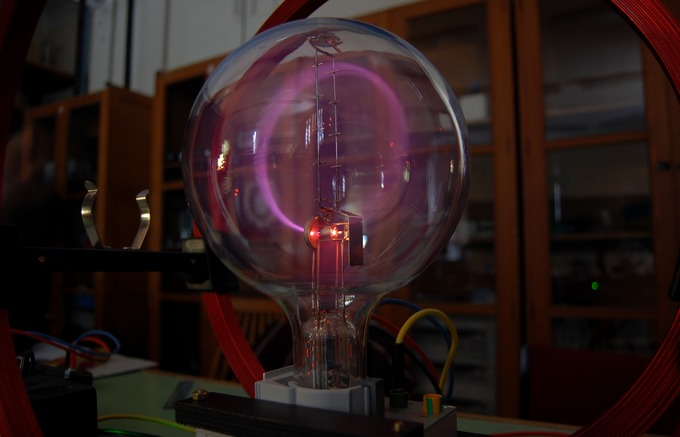Electron Beam: A beam of electrons deflected in a circle by a magnetic field.

### Proton

In 1917 (in experiments reported in 1919), Rutherford proved that the hydrogen nucleus is present in other nuclei, a result usually described as the discovery of the proton. Earlier, Rutherford learned to create hydrogen nuclei as a type of radiation produced as a yield of the impact of alpha particles on hydrogen gas; these nuclei were recognized by their unique penetration signature in air and their appearance in scintillation detectors. These experiments began when Rutherford noticed that when alpha particles were shot into air (mostly nitrogen), his scintillation detectors displayed the signatures of typical hydrogen nuclei as a product. After experimentation Rutherford traced the reaction to the nitrogen in air, and found that the effect was larger when alphas were produced into pure nitrogen gas. Rutherford determined that the only possible source of this hydrogen was the nitrogen, and therefore nitrogen must contain hydrogen nuclei. One hydrogen nucleus was knocked off by the impact of the alpha particle, producing oxygen-17 in the process. This was the first reported nuclear reaction, $14\text{N} + \alpha \rightarrow 17\text{O} + \text{p}$.

### Neutron

In 1920, Ernest Rutherford conceived the possible existence of the neutron. In particular, Rutherford examined the disparity found between the atomic number of an atom and its atomic mass. His explanation for this was the existence of a neutrally charged particle within the atomic nucleus. He considered the neutron to be a neutral double consisting of an electron orbiting a proton. In 1932, James Chadwick showed uncharged particles in the radiation he used. These particles had a similar mass as protons, but did not have the same characteristics as protons. Chadwick followed some of the predictions of Rutherford, the first to work in this then unknown field.

## Early Models of the Atom

Dalton believed that that matter is composed of discrete units called atoms — indivisible, ultimate particles of matter.

### Learning Objectives

Describe postulates of Dalton’s atomic theory and the atomic theories of ancient Greek philosophers

### Key Takeaways

#### Key Points

• The atom is a basic unit of matter that consists of a dense central nucleus surrounded by a cloud of negatively charged electrons.
• Scattered knowledge discovered by alchemists over the Middle Ages contributed to the discovery of atoms.
• Dalton established his atomic theory based on the fact that the masses of reactants in specific chemical reactions always have a particular mass ratio.

#### Key Terms

• electromagnetic force: a long-range fundamental force that acts between charged bodies, mediated by the exchange of photons
• Avogadro’s number: the number of constituent particles (usually atoms or molecules) in one mole of a given substance. It has dimensions of reciprocal mol and its value is equal to $6.02214129 \cdot 10^{23} \text{ mol}^{-1}$
• nucleus: the massive, positively charged central part of an atom, made up of protons and neutrons

The atom is a basic unit of matter that consists of a dense central nucleus surrounded by a cloud of negatively charged electrons. The atomic nucleus contains a mix of positively charged protons and electrically neutral neutrons (except in the case of hydrogen-1, which is the only stable nuclide with no neutrons). The electrons of an atom are bound to the nucleus by the electromagnetic force. We have a detailed (and accurate) model of the atom now, but it took a long time to come up with the correct answer.Illustration of the Helium Atom: This is an illustration of the helium atom, depicting the nucleus (pink) and the electron cloud distribution (black). The nucleus (upper right) in helium-4 is in reality spherically symmetric and closely resembles the electron cloud, although for more complicated nuclei this is not always the case. The black bar is one angstrom ($10^{-10}$ m, or 100 pm).

People have long speculated about the structure of matter and the existence of atoms. The earliest significant ideas to survive are from the ancient Greeks in the fifth century BC, especially from the philosophers Leucippus and Democritus. (There is some evidence that philosophers in both India and China made similar speculations at about the same time. ) They considered the question of whether a substance can be divided without limit into ever smaller pieces. There are only a few possible answers to this question. One is that infinitesimally small subdivision is possible. Another is what Democritus in particular believed — that there is a smallest unit that cannot be further subdivided. Democritus called this the atom. We now know that atoms themselves can be subdivided, but their identity is destroyed in the process, so the Greeks were correct in a respect. The Greeks also felt that atoms were in constant motion, another correct notion.

The Greeks and others speculated about the properties of atoms, proposing that only a few types existed and that all matter was formed as various combinations of these types. The famous proposal that the basic elements were earth, air, fire, and water was brilliant but incorrect. The Greeks had identified the most common examples of the four states of matter (solid, gas, plasma, and liquid) rather than the basic chemical elements. More than 2000 years passed before observations could be made with equipment capable of revealing the true nature of atoms.

Over the centuries, discoveries were made regarding the properties of substances and their chemical reactions. Certain systematic features were recognized, but similarities between common and rare elements resulted in efforts to transmute them (lead into gold, in particular) for financial gain. Secrecy was commonplace. Alchemists discovered and rediscovered many facts but did not make them broadly available. As the Middle Ages ended, the practice of alchemy gradually faded, and the science of chemistry arose. It was no longer possible, nor considered desirable, to keep discoveries secret. Collective knowledge grew, and by the beginning of the 19th century, an important fact was well established: the masses of reactants in specific chemical reactions always have a particular mass ratio. This is very strong indirect evidence that there are basic units (atoms and molecules) that have these same mass ratios. English chemist John Dalton (1766-1844) did much of this work, with significant contributions by the Italian physicist Amedeo Avogadro (1776-1856). It was Avogadro who developed the idea of a fixed number of atoms and molecules in a mole. This special number is called Avogadro’s number in his honor ($6.022 \cdot 10^{23}$).

Dalton believed that matter is composed of discrete units called atoms, as opposed to the obsolete notion that matter could be divided into any arbitrarily small quantity. He also believed that atoms are the indivisible, ultimate particles of matter. However, this belief was overturned near the end of the 19th century by Thomson, with his discovery of electrons.

Intro to the History of Atomic Theory – Intro: Rutherford, Thomson, electrons, nuclei, and plums. I don’t mean to be a bohr, but do you think pudding should have a role in serious scientific inquiry?

## The Thomson Model

Thomson proposed that the atom is composed of electrons surrounded by a soup of positive charge to balance the electrons’ negative charges.

### Learning Objectives

Describe model of an atom proposed by J. J. Thomson.

### Key Takeaways

#### Key Points

• J. J. Thomson, who discovered the electron in 1897, proposed the plum pudding model of the atom in 1904 before the discovery of the atomic nucleus in order to include the electron in the atomic model.
• In Thomson’s model, the atom is composed of electrons surrounded by a soup of positive charge to balance the electrons’ negative charges, like negatively charged “plums” surrounded by positively charged “pudding”.
• The 1904 Thomson model was disproved by Hans Geiger’s and Ernest Marsden’s 1909 gold foil experiment.

#### Key Terms

• nucleus: the massive, positively charged central part of an atom, made up of protons and neutrons

J. J. Thomson, who discovered the electron in 1897, proposed the plum pudding model of the atom in 1904 before the discovery of the atomic nucleus in order to include the electron in the atomic model. In Thomson’s model, the atom is composed of electrons (which Thomson still called “corpuscles,” though G. J. Stoney had proposed that atoms of electricity be called electrons in 1894) surrounded by a soup of positive charge to balance the electrons’ negative charges, like negatively charged “plums” surrounded by positively charged “pudding”. The electrons (as we know them today) were thought to be positioned throughout the atom in rotating rings. In this model the atom was also sometimes described to have a “cloud” of positive charge.Plum pudding model of the atom: A schematic presentation of the plum pudding model of the atom; in Thomson’s mathematical model the “corpuscles” (in modern language, electrons) were arranged non-randomly, in rotating rings.

With this model, Thomson abandoned his earlier “nebular atom” hypothesis, in which the atom was composed of immaterial vortices. Now, at least part of the atom was to be composed of Thomson’s particulate negative corpuscles, although the rest of the positively charged part of the atom remained somewhat nebulous and ill-defined.

The 1904 Thomson model was disproved by the 1909 gold foil experiment performed by Hans Geiger and Ernest Marsden. This gold foil experiment was interpreted by Ernest Rutherford in 1911 to suggest that there is a very small nucleus of the atom that contains a very high positive charge (in the case of gold, enough to balance the collective negative charge of about 100 electrons). His conclusions led him to propose the Rutherford model of the atom.

Intro to the History of Atomic Theory – The Thomson Model: Rutherford, Thomson, electrons, nuclei, and plums. I don’t mean to be a bohr, but do you think pudding should have a role in serious scientific inquiry?

## The Rutherford Model

Rutherford confirmed that the atom had a concentrated center of positive charge and relatively large mass.

### Learning Objectives

Describe gold foil experiment performed by Geiger and Marsden under directions of Rutherford and its implications for the model of the atom

### Key Takeaways

#### Key Points

• Rutherford overturned Thomson’s model in 1911 with his well-known gold foil experiment, in which he demonstrated that the atom has a tiny, high- mass nucleus.
• In his experiment, Rutherford observed that many alpha particles were deflected at small angles while others were reflected back to the alpha source.
• This highly concentrated, positively charged region is named the “nucleus” of the atom.

#### Key Terms

• alpha particle: A positively charged nucleus of a helium-4 atom (consisting of two protons and two neutrons), emitted as a consequence of radioactivity; α-particle.

The Rutherford model is a model of the atom named after Ernest Rutherford. Rutherford directed the famous Geiger-Marsden experiment in 1909, which suggested, according to Rutherford’s 1911 analysis, that J. J. Thomson’s so-called “plum pudding model” of the atom was incorrect. Rutherford’s new model for the atom, based on the experimental results, contained the new features of a relatively high central charge concentrated into a very small volume in comparison to the rest of the atom. This central volume also contained the bulk of the atom’s mass. This region would later be named the “nucleus. ”Atomic Planetary Model: Basic diagram of the atomic planetary model; electrons are in green, and the nucleus is in red

In 1911, Rutherford designed an experiment to further explore atomic structure using the alpha particles emitted by a radioactive element. Following his direction, Geiger and Marsden shot alpha particles with large kinetic energies toward a thin foil of gold. Measuring the pattern of scattered particles was expected to provide information about the distribution of charge within the atom. Under the prevailing plum pudding model, the alpha particles should all have been deflected by, at most, a few degrees. However, the actual results surprised Rutherford. Although many of the alpha particles did pass through as expected, many others were deflected at small angles while others were reflected back to the alpha source.

From purely energetic considerations of how far particles of known speed would be able to penetrate toward a central charge of 100 e, Rutherford was able to calculate that the radius of his gold central charge would need to be less than $3.4 \cdot 10^{-14}$ meters. This was in a gold atom known to be about $10^{-10}$ meters in radius; a very surprising finding, as it implied a strong central charge less than $\frac{1}{3000}$th of the diameter of the atom.

Intro to the History of Atomic Theory – The Rutherford Model: Rutherford, Thomson, electrons, nuclei, and plums. I don’t mean to be a bohr, but do you think pudding should have a role in serious scientific inquiry?

## The Bohr Model of the Atom

Bohr suggested that electrons in hydrogen could have certain classical motions only when restricted by a quantum rule.

### Learning Objectives

Describe model of atom proposed by Niels Bohr.

### Key Takeaways

#### Key Points

• According to Bohr: 1) Electrons in atoms orbit the nucleus, 2) The electrons can only orbit stably, without radiating, in certain orbits, and 3) Electrons can only gain and lose energy by jumping from one allowed orbit to another.
• The significance of the Bohr model is that the laws of classical mechanics apply to the motion of the electron about the nucleus only when restricted by a quantum rule. Therefore, his atomic model is called a semiclassical model.
• The laws of classical mechanics predict that the electron should release electromagnetic radiation while orbiting a nucleus, suggesting that all atoms should be unstable!

#### Key Terms

• Maxwell’s equations: A set of equations describing how electric and magnetic fields are generated and altered by each other and by charges and currents.
• semiclassical: a theory in which one part of a system is described quantum-mechanically whereas the other is treated classically.

### The Bohr Model of the Atom

The great Danish physicist Niels Bohr (1885–1962, ) made immediate use of Rutherford’s planetary model of the atom. Bohr became convinced of its validity and spent part of 1912 at Rutherford’s laboratory. In 1913, after returning to Copenhagen, he began publishing his theory of the simplest atom, hydrogen, based on the planetary model of the atom.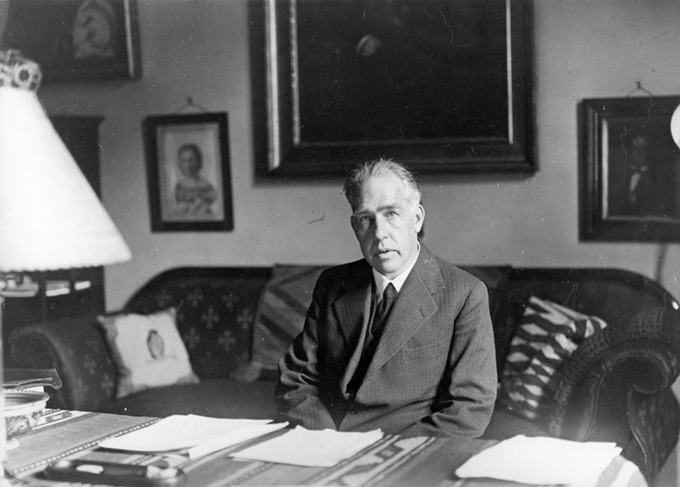Niels Bohr: Niels Bohr, Danish physicist, used the planetary model of the atom to explain the atomic spectrum and size of the hydrogen atom. His many contributions to the development of atomic physics and quantum mechanics; his personal influence on many students and colleagues; and his personal integrity, especially in the face of Nazi oppression, earned him a prominent place in history. (credit: Unknown Author, via Wikimedia Commons)

For decades, many questions had been asked about atomic characteristics. From their sizes to their spectra, much was known about atoms, but little had been explained in terms of the laws of physics. Bohr’s theory explained the atomic spectrum of hydrogen, made him instantly famous, and established new and broadly applicable principles in quantum mechanics.

One big puzzle that the planetary-model of atom had was the following. The laws of classical mechanics predict that the electron should release electromagnetic radiation while orbiting a nucleus (according to Maxwell’s equations, accelerating charge should emit electromagnetic radiation). Because the electron would lose energy, it would gradually spiral inwards, collapsing into the nucleus. This atom model is disastrous, because it predicts that all atoms are unstable. Also, as the electron spirals inward, the emission would gradually increase in frequency as the orbit got smaller and faster. This would produce a continuous smear, in frequency, of electromagnetic radiation. However, late 19th century experiments with electric discharges have shown that atoms will only emit light (that is, electromagnetic radiation) at certain discrete frequencies.

To overcome this difficulty, Niels Bohr proposed, in 1913, what is now called the Bohr model of the atom. He suggested that electrons could only have certain classical motions:

1. Electrons in atoms orbit the nucleus.
2. The electrons can only orbit stably, without radiating, in certain orbits (called by Bohr the “stationary orbits”): at a certain discrete set of distances from the nucleus. These orbits are associated with definite energies and are also called energy shells or energy levels. In these orbits, the electron’s acceleration does not result in radiation and energy loss as required by classical electrodynamics.
3. Electrons can only gain and lose energy by jumping from one allowed orbit to another, absorbing or emitting electromagnetic radiation with a frequency $\nu$ determined by the energy difference of the levels according to the Planck relation:

$\Delta{\text{E}} = \text{E}_2-\text{E}_1=\text{h}\nu \,$

where $\text{h}$ is Planck’s constant and $\nu$ is the frequency of the radiation.

### Semiclassical Model

The significance of the Bohr model is that the laws of classical mechanics apply to the motion of the electron about the nucleus only when restricted by a quantum rule. Therefore, his atomic model is called a semiclassical model.

## Basic Assumptions of the Bohr Model

Bohr explained hydrogen’s spectrum successfully by adopting a quantization condition and by introducing the Planck constant in his model.

### Learning Objectives

Describe basic assumptions that were applied by Niels Bohr to the planetary model of an atom

### Key Takeaways

#### Key Points

• Classical electrodynamics predicts that an atom described by a (classical) planetary model would be unstable.
• To explain the hydrogen spectrum, Bohr had to make a few assumptions that electrons could only have certain classical motions.
• After the seminal work by Planck, Einstein, and Bohr, physicists began to realize that it was essential to introduce the notion of ” quantization ” to explain microscopic worlds.

#### Key Terms

• black body: An idealized physical body that absorbs all incident electromagnetic radiation, regardless of frequency or angle of incidence. Although black body is a theoretical concept, you can find approximate realizations of black body in nature.
• photoelectric effect: The occurrence of electrons being emitted from matter (metals and non-metallic solids, liquids, or gases) as a consequence of their absorption of energy from electromagnetic radiation.

In previous modules, we have seen puzzles from classical atomic theories (e.g., the Rutherford model). Most importantly, classical electrodynamics predicts that an atom described by a (classical) planetary model would be unstable. To explain the puzzle, Bohr proposed what is now called the Bohr model of the atom in 1913. He suggested that electrons could only have certain classical motions:

1. Electrons in atoms orbit the nucleus.
2. The electrons can only orbit stably, without radiating, in certain orbits (called by Bohr the “stationary orbits”) at a certain discrete set of distances from the nucleus. These orbits are associated with definite energies and are also called energy shells or energy levels. In these orbits, the electron’s acceleration does not result in radiation and energy loss as required by classical electrodynamics.
3. Electrons can only gain and lose energy by jumping from one allowed orbit to another, absorbing or emitting electromagnetic radiation with a frequency $\nu$ determined by the energy difference of the levels according to the Planck relation: $\Delta{\text{E}} = \text{E}_2-\text{E}_1=\text{h}\nu$, where $\text{h}$ is the Planck constant. In addition, Bohr also assumed that the angular momentum $\text{L}$ is restricted to be an integer multiple of a fixed unit: $\text{L} = \text{n}{\text{h} \over 2\pi} = \text{n}\hbar$, where $\text{n} = 1, 2, 3, \dots$ is called the principal quantum number, and $\hbar = \frac{\text{h}}{2\pi}$.

We have seen that Planck adopted a new condition of energy quantization to explain the black body radiation, where he introduced the Planck constant $\text{h}$ for the first time. Soon after, Einstein resorted to this new concept of energy quantization and used the Planck constant again to explain the photoelectric effects, in which he assumed that electromagnetic radiation interact with matter as particles (later named “photons”). Here, Bohr explained the atomic hydrogen spectrum successfully for the first time by adopting a quantization condition and by introducing the Planck constant in his atomic model. Over the period of radical development in the early 20th century, physicists began to realize that it was essential to introduce the notion of “quantization” to explain microscopic worlds.Rutherford-Bohr model: The Rutherford–Bohr model of the hydrogen atom ($\text{Z}= 1$) or a hydrogen-like ion ($\text{Z}>1$), where the negatively charged electron confined to an atomic shell encircles a small, positively charged atomic nucleus, and where an electron jump between orbits is accompanied by an emitted or absorbed amount of electromagnetic energy ($\text{h}\nu$). The orbits in which the electron may travel are shown as gray circles; their radius increases as $\text{n}^2$, where $\text{n}$ is the principal quantum number. The $3 \rightarrow 2$ transition depicted here produces the first line of the Balmer series, and for hydrogen ($\text{Z}= 1$) it results in a photon of wavelength 656 nm (red light).

## Bohr Orbits

According to Bohr, electrons can only orbit stably, in certain orbits, at a certain discrete set of distances from the nucleus.

### Learning Objectives

Explain relationship between the “Bohr orbits” and the quantization effect

### Key Takeaways

#### Key Points

• The “Bohr orbits” have a very important feature of quantization: that the angular momentum L of an electron in its orbit is quantized, that is, it has only specific, discrete values. This leads to the equation L=mevrn=nℏL = m_e v r_n = n\hbar.
• At the time of proposal, Bohr himself did not know why angular momentum should be quantized, but using this assumption he was able to calculate the energies in the hydrogen spectrum.
• A theory of the atom or any other system must predict its energies based on the physics of the system, which the Bohr model was able to do.

#### Key Terms

• quantization: The process of explaining a classical understanding of physical phenomena in terms of a newer understanding known as quantum mechanics.

Danish Physicist Neils Bohr was clever enough to discover a method of calculating the electron orbital energies in hydrogen. As we’ve seen in the previous module “The Bohr Model of Atom,” Bohr assumed that the electrons can only orbit stably, without radiating, in certain orbits (named by Bohr as “stationary orbits”), at a certain discrete set of distances from the nucleus. These “Bohr orbits” have a very important feature of quantization as shown in the following. This was an important first step that has been improved upon, but it is well worth repeating here, as it correctly describes many characteristics of hydrogen. Assuming circular orbits, Bohr proposed that the angular momentum $\text{L}$ of an electron in its orbit is quantized, that is, has only specific, discrete values. The value for $\text{L}$ is given by the formula:

$\displaystyle \text{L} = \text{m}_\text{e} \text{v} \text{r}_\text{n} = \text{n}{\text{h} \over 2\pi} = \text{n}\hbar$

where $\text{L}$ is the angular momentum, $\text{m}_\text{e}$ is the electron’s mass, $\text{r}_\text{n}$ is the radius of the $\text{n}$-th orbit, and $\text{h}$ is Planck’s constant. Note that angular momentum is $\text{L} = \text{I}\omega$. For a small object at a radius $\text{r}$, $\text{I} = \text{mr}^2$ and $\omega = \frac{\text{v}}{\text{r}}$, so that:

$\displaystyle \text{L} = (\text{mr}^2)(\frac{\text{v}}{\text{r}}) = \text{mvr}$

Quantization says that this value of $\text{mvr}$ can only have discrete values. At the time, Bohr himself did not know why angular momentum should be quantized, but using this assumption he was able to calculate the energies in the hydrogen spectrum, something no one else had done at the time.

Below is an energy-level diagram, which is a convenient way to display energy states—the allowed energy levels of the electron (as relative to our discussion). Energy is plotted vertically with the lowest or ground state at the bottom and with excited states above. Given the energies of the lines in an atomic spectrum, it is possible (although sometimes very difficult) to determine the energy levels of an atom. Energy-level diagrams are used for many systems, including molecules and nuclei. A theory of the atom or any other system must predict its energies based on the physics of the system.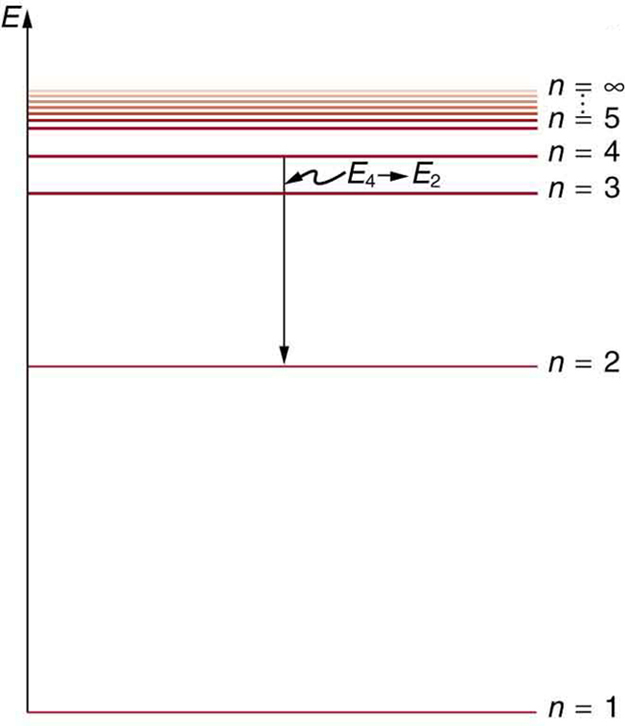Energy-Level Diagram Plot: An energy-level diagram plots energy vertically and is useful in visualizing the energy states of a system and the transitions between them. This diagram is for the hydrogen-atom electrons, showing a transition between two orbits having energies $\text{E}_4$ and $\text{E}_2$.

## Energy of a Bohr Orbit

Based on his assumptions, Bohr derived several important properties of the hydrogen atom from the classical physics.

### Learning Objectives

Apply proper equation to calculate energy levels and the energy of an emitted photon for a hydrogen-like atom

### Key Takeaways

#### Key Points

• According to Bohr, allowed orbit radius at any $\text{n}$ is $\text{r}_\text{n} = {\text{n}^2\hbar^2\over \text{Zk}_\text{e} \text{e}^2 \text{m}_\text{e}}$. The smallest possible value of $\text{r}$ in the hydrogen atom is called the Bohr radius and is equal to 0.053 nm.
• The energy of the $\text{n}$-th level for any atom is $\text{E} = \approx {-13.6\text{Z}^2 \over \text{n}^2}\mathrm{\text{eV}}$.
• The energy of a photon emitted by a hydrogen atom is given by the difference of two hydrogen energy levels: $\text{E}=\text{E}_\text{i}-\text{E}_\text{f}=\text{R} \left( \frac{1}{\text{n}_{\text{f}}^2} - \frac{1}{\text{n}_{\text{i}}^2} \right) \,$, which is known as Rydberg formula.

#### Key Terms

• centripetal: Directed or moving towards a center.

From Bohr’s assumptions, we will now derive a number of important properties of the hydrogen atom from the classical physics. We start by noting the centripetal force causing the electron to follow a circular path is supplied by the Coulomb force. To be more general, we note that this analysis is valid for any single-electron atom. So, if a nucleus has $\text{Z}$ protons ($\text{Z}=1$ for hydrogen, $\text{Z}=2$ for helium, etc.) and only one electron, that atom is called a hydrogen-like atom.

The spectra of hydrogen-like ions are similar to hydrogen, but shifted to higher energy by the greater attractive force between the electron and nucleus. The magnitude of the centripetal force is $\frac{\text{m}_\text{ev}^2}{\text{r}_\text{n}}$, while the Coulomb force is $\frac{\text{Zk}_\text{e} \text{e}^2}{\text{r}^2}$. The tacit assumption here is that the nucleus is more massive than the stationary electron, and the electron orbits about it. This is consistent with the planetary model of the atom. Equating these:

$\displaystyle \frac{\text{m}_\text{e} \text{v}^2}{\text{r}} = \frac{\text{Zk}_\text{e} \text{e}^2}{\text{r}^2}$

This equation determines the electron’s speed at any radius:

$\displaystyle \text{v} = \frac{\sqrt{\text{Zk}_\text{e} \text{e}^2}}{\text{m}_\text{e} \text{r}}$

It also determines the electron’s total energy at any radius:

$\displaystyle \text{E}= \frac{1}{2} \text{m}_\text{e} \text{v}^2 - \frac{\text{Z} \text{k}_\text{e} \text{e}^2}{\text{r}} = - \frac{\text{Z} \text{k}_\text{e} \text{e}^2}{2\text{r}}$

The total energy is negative and inversely proportional to $\text{r}$. This means that it takes energy to pull the orbiting electron away from the proton. For infinite values of $\text{r}$, the energy is zero, corresponding to a motionless electron infinitely far from the proton.

Now, here comes the Quantum rule: As we saw in the previous module, the angular momentum $\text{L} = \text{m}_\text{e} \text{r} \text{v}$ is an integer multiple of $\hbar$:

$\text{m}_\text{e} \text{v} \text{r} = \text{n} \hbar$

Substituting the expression in the equation for speed above gives an equation for $\text{r}$ in terms of $\text{n}$:

$\sqrt{\text{Zk}_\text{e} \text{e}^2 \text{m}_\text{e} \text{r}} = \text{n} \hbar$

The allowed orbit radius at any n is then:

$\displaystyle \text{r}_\text{n} = {\text{n}^2\hbar^2\over \text{Zk}_\text{e} \text{e}^2 \text{m}_\text{e}}$

The smallest possible value of $\text{r}$ in the hydrogen atom is called the Bohr radius and is equal to 0.053 nm. The energy of the $\text{n}$-th level for any atom is determined by the radius and quantum number:

$\displaystyle \text{E} = -\frac{\text{Zk}_\text{e} \text{e}^2}{2\text{r}_\text{n} } = - \frac{ \text{Z}^2(\text{k}_\text{e} \text{e}^2)^2 \text{m}_\text{e} }{2\hbar^2 \text{n}^2} \approx \frac{-13.6\text{Z}^2}{\text{n}^2}\mathrm{\text{eV}}$

Using this equation, the energy of a photon emitted by a hydrogen atom is given by the difference of two hydrogen energy levels:

$\displaystyle \text{E}=\text{E}_\text{i}-\text{E}_\text{f}=\text{R} \left( \frac{1}{\text{n}_{\text{f}}^2} - \frac{1}{\text{n}_{\text{i}}^2} \right)$

Which is the Rydberg formula describing all the hydrogen spectrum and $\text{R}$ is the Rydberg constant. Bohr’s model predicted experimental hydrogen spectrum extremely well.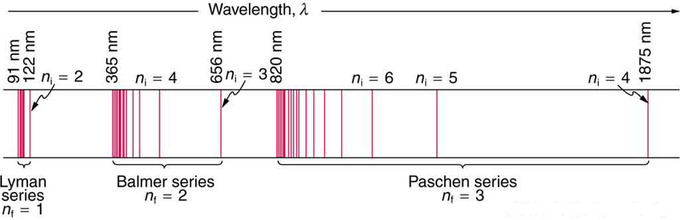Fig 1: A schematic of the hydrogen spectrum shows several series named for those who contributed most to their determination. Part of the Balmer series is in the visible spectrum, while the Lyman series is entirely in the UV, and the Paschen series and others are in the IR. Values of nf and ni are shown for some of the lines.

## Hydrogen Spectra

The observed hydrogen-spectrum wavelengths can be calculated using the following formula: $\frac{1}{\lambda} = \text{R}(\frac{1}{\text{n}_\text{f} ^2} - \frac{1}{\text{n}_\text{i} ^2})$.

### Learning Objectives

Explain difference between Lyman, Balmer, and Paschen series

### Key Takeaways

#### Key Points

• Atomic and molecular emission and absorption spectra have been known for over a century to be discrete (or quantized).
• Lyman, Balmer, and Paschen series are named after early researchers who studied them in particular depth.
• Bohr was the first one to provide a theoretical explanation of the hydrogen spectra.

#### Key Terms

• photon: The quantum of light and other electromagnetic energy, regarded as a discrete particle having zero rest mass, no electric charge, and an indefinitely long lifetime.
• spectrum: A condition that is not limited to a specific set of values but can vary infinitely within a continuum. The word saw its first scientific use within the field of optics to describe the rainbow of colors in visible light when separated using a prism.

For decades, many questions had been asked about atomic characteristics. From their sizes to their spectra, much was known about atoms, but little had been explained in terms of the laws of physics. Atomic and molecular emission and absorption spectra have been known for over a century to be discrete (or quantized). Maxwell and others had realized that there must be a connection between the spectrum of an atom and its structure, something like the resonant frequencies of musical instruments. But, despite years of efforts by many great minds, no one had a workable theory. (It was a running joke that any theory of atomic and molecular spectra could be destroyed by throwing a book of data at it, so complex were the spectra.) Following Einstein’s proposal of photons with quantized energies directly proportional to their wavelengths, it became even more evident that electrons in atoms can exist only in discrete orbits.

In some cases, it had been possible to devise formulas that described the emission spectra. As you might expect, the simplest atom—hydrogen, with its single electron—has a relatively simple spectrum. The hydrogen spectrum had been observed in the infrared (IR), visible, and ultraviolet (UV), and several series of spectral lines had been observed. The observed hydrogen-spectrum wavelengths can be calculated using the following formula:

$\displaystyle \frac{1}{\lambda} = \text{R}(\frac{1}{\text{n}_\text{f} ^2} - \frac{1}{\text{n}_\text{i} ^2})$

where $\lambda$ is the wavelength of the emitted EM radiation and $\text{R}$ is the Rydberg constant, determined by the experiment to be $\text{R}=1.097\cdot 10^7 \text{\text{m}}^{-1}$, and $\text{n}_\text{f}$, $\text{n}_\text{i}$ are positive integers associated with a specific series.

These series are named after early researchers who studied them in particular depth. For the Lyman series, $\text{n}_\text{f} = 1$ for the Balmer series, $\text{n}_\text{f} = 2$; for the Paschen series, $\text{n}_\text{f} = 3$; and so on. The Lyman series is entirely in the UV, while part of the Balmer series is visible with the remainder UV. The Paschen series and all the rest are entirely IR. There are apparently an unlimited number of series, although they lie progressively farther into the infrared and become difficult to observe as $\text{n}_\text{f}$ increases. The constant $\text{n}_\text{i}$ is a positive integer, but it must be greater than $\text{n}_\text{f}$. Thus, for the Balmer series, $\text{n}_\text{f} = 2$ and $\text{n}_\text{i} = 3,4,5,6…$. Note that $\text{n}_\text{i}$ can approach infinity.Electron transitions and their resulting wavelengths for hydrogen.: Energy levels are not to scale.

While the formula in the wavelengths equation was just a recipe designed to fit data and was not based on physical principles, it did imply a deeper meaning. Balmer first devised the formula for his series alone, and it was later found to describe all the other series by using different values of $\text{n}_\text{f}$. Bohr was the first to comprehend the deeper meaning. Again, we see the interplay between experiment and theory in physics. Experimentally, the spectra were well established, an equation was found to fit the experimental data, but the theoretical foundation was missing.

## de Broglie and the Bohr Model

By assuming that the electron is described by a wave and a whole number of wavelengths must fit, we derive Bohr’s quantization assumption.

### Learning Objectives

Describe reinterpretation of Bohr’s condition by de Broglie

### Key Takeaways

#### Key Points

• Bohr’s condition, that the angular momentum is an integer multiple of $\hbar$, was later reinterpreted in 1924 by de Broglie as a standing wave condition.
• For what Bohr was forced to hypothesize as the rule for allowed orbits, de Broglie’s matter wave concept explains it as the condition for constructive interference of an electron in a circular orbit.
• Bohr’s model was only applicable to hydrogen-like atoms. In 1925, more general forms of description (now called quantum mechanics ) emerged, thanks to Heisenberg and Schrodinger.

#### Key Terms

• standing wave: A wave form which occurs in a limited, fixed medium in such a way that the reflected wave coincides with the produced wave. A common example is the vibration of the strings on a musical stringed instrument.
• matter wave: A concept reflects the wave-particle duality of matter. The theory was proposed by Louis de Broglie.

Bohr’s condition, that the angular momentum is an integer multiple of $\hbar$, was later reinterpreted in 1924 by de Broglie as a standing wave condition. The wave-like properties of matter were subsequently confirmed by observations of electron interference when scattered from crystals. Electrons can exist only in locations where they interfere constructively. How does this affect electrons in atomic orbits? When an electron is bound to an atom, its wavelength must fit into a small space, something like a standing wave on a string.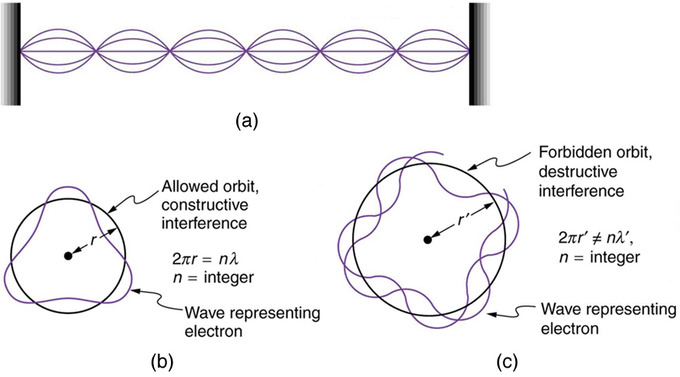Waves on a String: (a) Waves on a string have a wavelength related to the length of the string, allowing them to interfere constructively. (b) If we imagine the string bent into a closed circle, we get a rough idea of how electrons in circular orbits can interfere constructively. (c) If the wavelength does not fit into the circumference, the electron interferes destructively; it cannot exist in such an orbit.

Allowed orbits are those in which an electron constructively interferes with itself. Not all orbits produce constructive interference and thus only certain orbits are allowed (i.e., the orbits are quantized). By assuming that the electron is described by a wave and a whole number of wavelengths must fit along the circumference of the electron’s orbit, we have the equation:

$\text{n}\lambda =2\pi \text{r}$

Substituting de Broglie’s wavelength of $\frac{\text{h}}{\text{p}}$ reproduces Bohr’s rule. Since $\lambda = \text{h}/\text{m}_\text{ev}$, we now have:

$\displaystyle \frac{\text{nh}}{\text{m}_\text{ev}} = 2\pi \text{r}_\text{n}$

Rearranging terms, and noting that $\text{L}=\text{mvr}$ for a circular orbit, we obtain the quantization of angular momentum as the condition for allowed orbits:

$\displaystyle \text{L} = \text{m}_\text{e} \text{v} \text{r}_\text{n} = \text{n} \frac{\text{h}}{2\pi}, (\text{n}=1,2,3…)$

As previously stated, Bohr was forced to hypothesize this rule for allowed orbits. We now realize this as the condition for constructive interference of an electron in a circular orbit.

Accordingly, a new kind of mechanics, quantum mechanics, was proposed in 1925. Bohr’s model of electrons traveling in quantized orbits was extended into a more accurate model of electron motion. The new theory was proposed by Werner Heisenberg. By different reasoning, another form of the same theory, wave mechanics, was discovered independently by Austrian physicist Erwin Schrödinger. Schrödinger employed de Broglie’s matter waves, but instead sought wave solutions of a three-dimensional wave equation. This described electrons that were constrained to move about the nucleus of a hydrogen-like atom by being trapped by the potential of the positive nuclear charge.

de Broglie’s Matter Waves Justify Bohr’s Magic Electron Orbital Radii: I include a summary of the hydrogen atom’s electronic structure and explain how an electron can interfere with itself in an orbit just like it can in a double-slit experiment.

## X-Rays and the Compton Effect

Compton explained the X-ray frequency shift during the X-ray/electron scattering by attributing particle-like momentum to “photons”.

### Learning Objectives

Describe Compton effects between electrons and x-ray photons

### Key Takeaways

#### Key Points

• Compton derived the mathematical relationship between the shift in wavelength and the scattering angle of the X-rays.
• Compton effects (with electrons) usually occur with x-ray photons.
• If the photon is of lower energy, in the visible light through soft X-rays range, photoelectric effects are observed. Higher energy photons, in the gamma ray range, may lead to pair production.

#### Key Terms

• gamma ray: A very high frequency (and therefore very high energy) electromagnetic radiation emitted as a consequence of radioactivity.
• photoelectric effects: In photoelectric effects, electrons are emitted from matter (metals and non-metallic solids, liquids or gases) as a consequence of their absorption of energy from electromagnetic radiation.
• photon: The quantum of light and other electromagnetic energy, regarded as a discrete particle having zero rest mass, no electric charge, and an indefinitely long lifetime.

By the early 20th century, research into the interaction of X-rays with matter was well underway. It was observed that when X-rays of a known wavelength interact with atoms, the X-rays are scattered through an angle $\theta$ and emerge at a different wavelength related to $\theta$. Although classical electromagnetism predicted that the wavelength of scattered rays should be equal to the initial wavelength, multiple experiments had found that the wavelength of the scattered rays was longer (corresponding to lower energy) than the initial wavelength.

In 1923, Compton published a paper in the Physical Review which explained the X-ray shift by attributing particle-like momentum to “photons,” which Einstein had invoked in his Nobel prize winning explanation of the photoelectric effect. First postulated by Planck, these “particles” conceptualized “quantized” elements of light as containing a specific amount of energy depending only on the frequency of the light. In his paper, Compton derived the mathematical relationship between the shift in wavelength and the scattering angle of the X-rays by assuming that each scattered X-ray photon interacted with only one electron. His paper concludes by reporting on experiments which verified his derived relation:

$\displaystyle \lambda' - \lambda = \frac{\text{h}}{\text{m}_\text{e} \text{c}}(1-\cos{\theta})$

where λ\lambda is the initial wavelength, λ′\lambda’ is the wavelength after scattering, $\text{h}$ is the Planck constant, mem_e is the Electron rest mass, $\text{c}$ is the speed of light, and θ\theta is the scattering angle. The quantity hmec\frac{h}{m_e c} is known as the Compton wavelength of the electron; it is equal to $2.43\cdot 10^{-12}$ m. The wavelength shift $\lambda ‘ - \lambda$ is at least zero (for $\theta = 0$°) and at most twice the Compton wavelength of the electron (for $\theta = 180$°). (The derivation of Compton’s formula is a bit lengthy and will not be covered here.)A Photon Colliding with a Target at Rest: A photon of wavelength $\lambda$ comes in from the left, collides with a target at rest, and a new photon of wavelength $\lambda ‘$ emerges at an angle $\theta$.

Because the mass-energy and momentum of a system must both be conserved, it is not generally possible for the electron simply to move in the direction of the incident photon. The interaction between electrons and high energy photons (comparable to the rest energy of the electron, 511 keV) results in the electron being given part of the energy (making it recoil), and a photon containing the remaining energy being emitted in a different direction from the original, so that the overall momentum of the system is conserved. If the scattered photon still has enough energy left, the Compton scattering process may be repeated. In this scenario, the electron is treated as free or loosely bound. Photons with an energy of this order of magnitude are in the x-ray range of the electromagnetic radiation spectrum. Therefore, you can say that Compton effects (with electrons) occur with x-ray photons.

If the photon is of lower energy, but still has sufficient energy (in general a few eV to a few keV, corresponding to visible light through soft X-rays), it can eject an electron from its host atom entirely (a process known as the photoelectric effect), instead of undergoing Compton scattering. Higher energy photons (1.022 MeV and above, in the gamma ray range) may be able to bombard the nucleus and cause an electron and a positron to be formed, a process called pair production.

## X-Ray Spectra: Origins, Diffraction by Crystals, and Importance

X-ray shows its wave nature when radiated upon atomic/molecular structures and can be used to study them.

### Learning Objectives

Describe interactions between X-rays and atoms

### Key Takeaways

#### Key Points

• X rays are relatively high- frequency EM radiation. They are produced by transitions between inner-shell electron levels, which produce x rays characteristic of the atomic element, or by accelerating electrons.
• x-ray diffraction is a technique that provides the detailed information about crystallographic structure of natural and manufactured materials.
• Current research in material science and physics involves complex materials whose lattice arrangements are crucial to obtaining a superconducting material, which can be studied using x-ray crystallography.

#### Key Terms

• double-helix structure: The structure formed by double-stranded molecules of nucleic acids such as DNA and RNA.
• crystallography: The experimental science of determining the arrangement of atoms in solids.
• diffraction: The bending of a wave around the edges of an opening or an obstacle.

In a previous Atom on X-rays, we have seen that there are two processes by which x-rays are produced in the anode of an x-ray tube. In one process, the deceleration of electrons produces x-rays, and these x-rays are called Bremsstrahlung, or braking radiation. The second process is atomic in nature and produces characteristic x-rays, so called because they are characteristic of the anode material. The x-ray spectrum in is typical of what is produced by an x-ray tube, showing a broad curve of Bremsstrahlung radiation with characteristic x-ray peaks on it.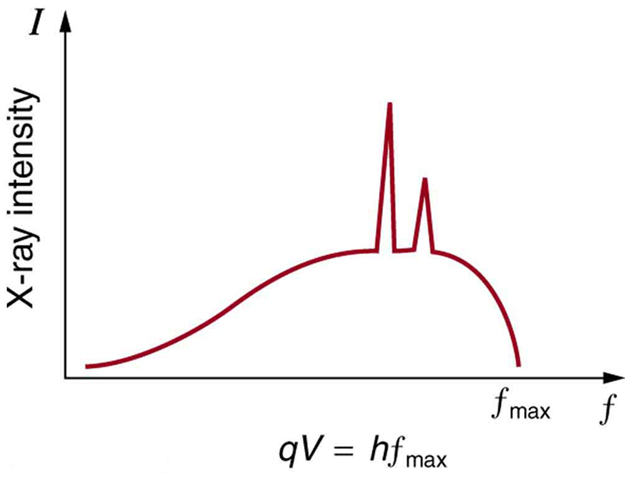X-Ray Spectrum: X-ray spectrum obtained when energetic electrons strike a material, such as in the anode of a CRT. The smooth part of the spectrum is bremsstrahlung radiation, while the peaks are characteristic of the anode material. A different anode material would have characteristic x-ray peaks at different frequencies.

Since x-ray photons are very energetic, they have relatively short wavelengths. For example, the 54.4-keV Kα x-ray, for example, has a wavelength $\lambda = \frac{\text{hc}}{\text{E}} = 0.0228$ nm. Thus, typical x-ray photons act like rays when they encounter macroscopic objects, like teeth, and produce sharp shadows. However, since atoms and atomic structures have a typical size on the order of 0.1 nm, x-ray shows its wave nature with them. The process is called x-ray diffraction because it involves the diffraction and interference of x-rays to produce patterns that can be analyzed for information about the structures that scattered the x-rays.

Shown below, Bragg’s Law gives the angles for coherent and incoherent scattering of light from a crystal lattice, which happens during x-ray diffraction. When x-ray are incident on an atom, they make the electronic cloud move as an electromagnetic wave. The movement of these charges re-radiate waves with the same frequency. This is called Rayleigh Scattering, which you should remember from a previous atom. A similar thing happens when neutron waves from the nuclei scatter from interaction with an unpaired electron. These re-emitted wave fields interfere with each other either constructively or destructively, and produce a diffraction pattern that is captured by a sensor or film. This is called the Braggs diffraction, and is the basis for x-ray diffraction.X-Ray Diffraction: Bragg’s Law of diffraction: illustration of how x-rays interact with crystal lattice.

Perhaps the most famous example of x-ray diffraction is the discovery of the double-helix structure of DNA in 1953. Using x-ray diffraction data, researchers were able to discern the structure of DNA shows a diffraction pattern produced by the scattering of x-rays from a crystal of protein. This process is known as x-ray crystallography because of the information it can yield about crystal structure. Not only do x-rays confirm the size and shape of atoms, they also give information on the atomic arrangements in materials. For example, current research in high-temperature superconductors involves complex materials whose lattice arrangements are crucial to obtaining a superconducting material. These can be studied using x-ray crystallography.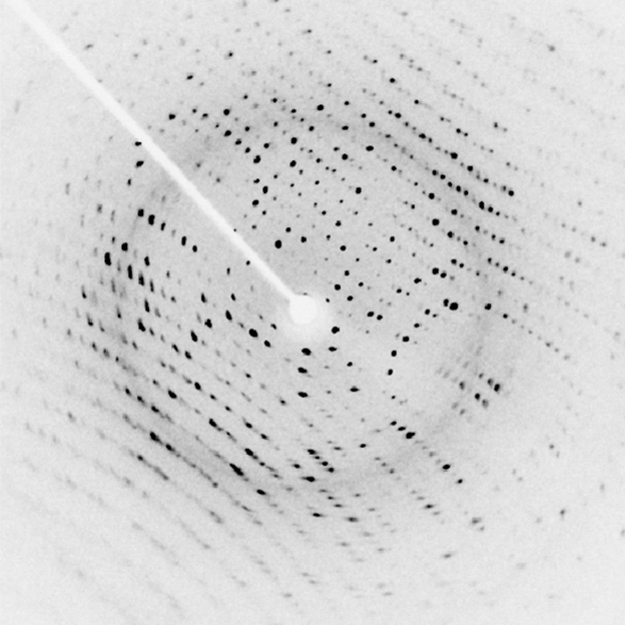X-Ray Diffraction: X-ray diffraction from the crystal of a protein, hen egg lysozyme, produced this interference pattern. Analysis of the pattern yields information about the structure of the protein.

## The Compton Effect

The Compton Effect is the phenomenon of the decrease in energy of photon when scattered by a free charged particle.

### Learning Objectives

Explain why Compton scattering is an inelastic scattering.

### Key Takeaways

#### Key Points

• Compton scattering is an example of inelastic scattering because the wavelength of the scattered light is different from the incident radiation.
• Like the photoelectric effects, the Compton effect is important because it demonstrates that light cannot be explained purely as a wave phenomenon. Light must behave as if it consists of particles to explain the Compton scattering.
• Compton’s experiment convinced physicists that light can behave as a stream of particle-like objects (quanta) whose energy is proportional to the frequency.

#### Key Terms

• Doppler shift: is the change in frequency of a wave for an observer moving relative to its source.
• Thomson scattering: an elastic scattering of electromagnetic radiation by a free charged particle, as described by classical electromagnetism. It is just the low-energy limit of Compton scattering
• inelastic scattering: a fundamental scattering process in which the kinetic energy of an incident particle is not conserved

Compton scattering is an inelastic scattering of a photon by a free charged particle (usually an electron). It results in a decrease in energy (increase in wavelength) of the photon (which may be an X-ray or gamma ray photon), called the Compton Effect. Part of the energy of the photon is transferred to the scattering electron. Inverse Compton scattering also exists, and happens when a charged particle transfers part of its energy to a photon.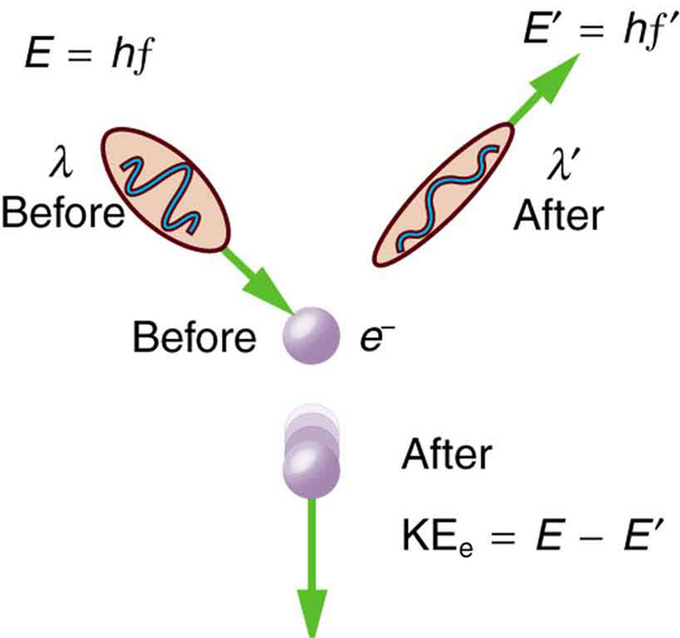Scattering in the Compton Effect: The Compton Effect is the name given to the scattering of a photon by an electron. Energy and momentum are conserved, resulting in a reduction of both for the scattered photon. Studying this effect, Compton verified that photons have momentum.

Compton scattering is an example of inelastic scattering because the wavelength of the scattered light is different from the incident radiation. Still, the origin of the effect can be considered as an elastic collision between a photon and an electron. The amount of change in the wavelength is called the Compton shift. Although nuclear Compton scattering exists, Compton scattering usually refers to the interaction involving only the electrons of an atom.

The Compton effect is important because it demonstrates that light cannot be explained purely as a wave phenomenon. Thomson scattering, the classical theory of an electromagnetic wave scattered by charged particles, cannot explain low intensity shifts in wavelength: classically, light of sufficient intensity for the electric field to accelerate a charged particle to a relativistic speed will cause radiation-pressure recoil and an associated Doppler shift of the scattered light. However, the effect will become arbitrarily small at sufficiently low light intensities regardless of wavelength. Light must behave as if it consists of particles to explain the low-intensity Compton scattering. Compton’s experiment convinced physicists that light can behave as a stream of particle-like objects (quanta) whose energy is proportional to the frequency.